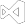# Read Excel Files without InteropRead Excel Files without Interop
``````using IronXL;
using System.Linq;

//Supported spreadsheet formats for reading include: XLSX, XLS, CSV and TSV
WorkBook workbook = WorkBook.Load("test.xlsx");
WorkSheet sheet = workbook.WorkSheets.First();

//Select cells easily in Excel notation and return the calculated value
int cellValue = sheet["A2"].IntValue;

// Read from Ranges of cells elegantly.
foreach (var cell in sheet["A2:A10"])
{
Console.WriteLine("Cell {0} has value '{1}'", cell.AddressString, cell.Text);
}

//Calculate aggregate values such as Min, Max and Sum
decimal sum = sheet["A2:A10"].Sum();

//Linq compatible
decimal max = sheet["A2:A10"].Max(c => c.DecimalValue);``````
``````Imports IronXL
Imports System.Linq

'Supported spreadsheet formats for reading include: XLSX, XLS, CSV and TSV
Private workbook As WorkBook = WorkBook.Load("test.xlsx")
Private sheet As WorkSheet = workbook.WorkSheets.First()

'Select cells easily in Excel notation and return the calculated value
Private cellValue As Integer = sheet("A2").IntValue

' Read from Ranges of cells elegantly.
For Each cell In sheet("A2:A10")
Console.WriteLine("Cell {0} has value '{1}'", cell.AddressString, cell.Text)
Next cell

'Calculate aggregate values such as Min, Max and Sum
Dim sum As Decimal = sheet("A2:A10").Sum()

'Linq compatible
Dim max As Decimal = sheet("A2:A10").Max(Function(c) c.DecimalValue)``````

IronXL is an Excel Library for C# and .Net which allows developers to Read and edit Excel data from XLS and XLSX Documents without using Microsoft.Office.Interop.Excel.

The API allows us to Create, Read, Manipulate, Save and Export Excel files intuitively for:

• .Net Framework 4.5+
• .Net Core 2+
• .Net Standard
• Xamarin
• Windows Mobile
• Mono
• & Azure Cloud hosting
IronXL also fully supports ASP.Net, MVC, Windows, MacOS, Linux, iOS, Android and Windows Mobile application development.